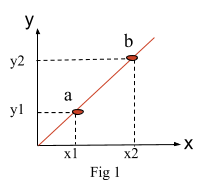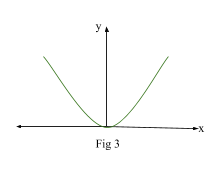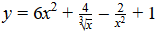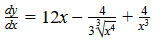# Differentiation

Contents

## Summary

• Differentiation is a method to find the gradient of a curve.
• When differentiating a function, always remember to rewrite the equation as a power of x. This makes it easier to differentiate.
• Differentiation formula: if$\quad y\quad =\quad { x }^{ n }$, where n is a real constant. Then$\quad \frac { dy }{ dx } \quad =\quad n{ x }^{ n\quad -\quad 1 }$.
• Derivative of a constant is always 0.
• Basic Rules of Differentiation:
1. If$\quad y\quad =\quad { x }^{ n }$, then$\quad \frac { dy }{ dx } \quad =\quad n{ x }^{ n\quad -\quad 1 }$
2. If y = k, where k is a constant, then$\quad \frac { dy }{ dx } \quad =\quad 0$
3. If$\quad y\quad =\quad k{ x }^{ n }$, where k is a constant, then$\quad \frac { dy }{ dx } \quad =\quad kn{ x }^{ n\quad -\quad 1 }$
4. If$\quad y\quad =\quad f\left( x \right)\quad \pm \quad g\left( x \right)$ (sum or difference of two functions), then$\quad \frac { dy }{ dx } \quad =\quad f^{ ' }\left( x \right) \quad \pm \quad g^{ ' }\left( x \right)$

#### What is Differentiation?

Differentiation is the process that we use to find the gradient of a point on the curve.

To understand this better we would recall that to find the gradient (slope) of a straight line, we simply divide the change in y by the change in x.

E.g We know that slope of a straight line is always constant. The slope basically characterizes the direction of the line.

We find the slope by first choosing any two points (a & b) on the line as shown in ‘Fig 1’.We then use the following formula to find the slope:$Slope\quad =\quad \frac { { y }_{ 2 }\quad -\quad { y }_{ 1 } }{ { x }_{ 2 }\quad -\quad { x }_{ 1 } }$

However, a curve is not a straight line, hence its gradient is not constant. It is changing from one point to another point on the curve. So we don’t have a set gradient for a curve.

We define a gradient function as$\frac { dy }{ dx }$ and call it a derivative or differential coefficient of y with respect to x. The value of$\frac { dy }{ dx }$ at a given point (eg point ‘p’ in ‘Fig 2’) is the gradient of the curve and hence it is also the gradient of the tangent at that point as shown in ‘Fig 2’. This applies to any point along the curve.Working out the gradient at a point also tells us the rate of change of the curve at that point.

E.g from a velocity vs time graphs we can work out the acceleration as the rate of of change of velocity with respect to time is the acceleration.

Recall: A tangent is a line that just touches a curve without actually going through it.

#### How do we differentiate?

To find the derivative of a given function we use the following formula:

If$y\quad =\quad { x }^{ n }$, where n is a real constant. Then$\frac { dy }{ dx } \quad =\quad n{ x }^{ n\quad -\quad 1 }$

#### Example #1

We start of with a simple example first.

You need the gradient of the graph of$y\quad =\quad { x }^{ 2 }$ at the point x = 1. Graph is shown in ‘Fig 3’.Now differentiate the function using the above formula.$y\quad =\quad { x }^{ 2 }$

Differentiating y with respect to x:$\frac { dy }{ dx } \quad =\quad 2x$      (Derivative)

To find the gradient at x = 1 substitute in above equation.$\frac { dy }{ dx } \quad =\quad 2$

Hence:

Gradient of the graph at x = 1 is 2.

Tip: It is much easier to differentiate when the equations are written as power of x.

In a case where there are more terms in an equation, each term should be differentiated separately.

#### Example #2

If you have a function:Remember: Derivative of a constant is always 0.

We first write the terms as power of x:$=\quad 6{ x }^{ 2 }\quad +\quad 4{ x }^{ -\frac { 1 }{ 3 } }\quad -\quad 2{ x }^{ -2 }\quad +\quad 1$

Next we find the derivative of the whole function, but differentiating each term separately.$\frac { dy }{ dx } \quad =\quad 6(2x)\quad +\quad 4(-\frac { 1 }{ 3 } { x }^{ -\frac { 4 }{ 3 } })\quad -\quad 2(-2{ x }^{ -3 })\quad +\quad 0$

After simplifying:(Derivative)

#### Basic rules of Differentiation:

1.  If$y\quad =\quad { x }^{ n }$ , then$\frac { dy }{ dx } \quad =\quad n{ x }^{ n\quad -\quad 1 }$

E.g if$y\quad =\quad { x }^{ 10\quad }$ , then$\frac { dy }{ dx } \quad =\quad 10{ x }^{ 9\quad }$

2.  If y = k, where k is a constant, then$\quad \frac { dy }{ dx } \quad =\quad 0$

E.g if y = 10, then$\frac { dy }{ dx } \quad =\quad 0$

3.  If$\quad y\quad =\quad k{ x }^{ n }$,  where k is a constant, then$\quad \frac { dy }{ dx } \quad =\quad kn{ x }^{ n\quad -\quad 1 }$

E.g if$y\quad =\quad 5{ x }^{ 2 }$,  then$\frac { dy }{ dx } \quad =\quad 10$

4.  If$\quad y\quad =\quad f\left( x \right)\quad \pm \quad g\left( x \right)$  (sum or difference of two functions), then$\quad \frac { dy }{ dx } \quad =\quad f^{ ' }\left( x \right) \quad \pm \quad g^{ ' }\left( x \right)$

E.g if$y\quad =\quad { x }^{ 2 }\quad +\quad { x }^{ 4 }$,  then$\frac { dy }{ dx } \quad =\quad 2x\quad +\quad 4{ x }^{ 3 }$

###### Reference
1. CGP Mathematics Edexcel Core 1 Revision Guide